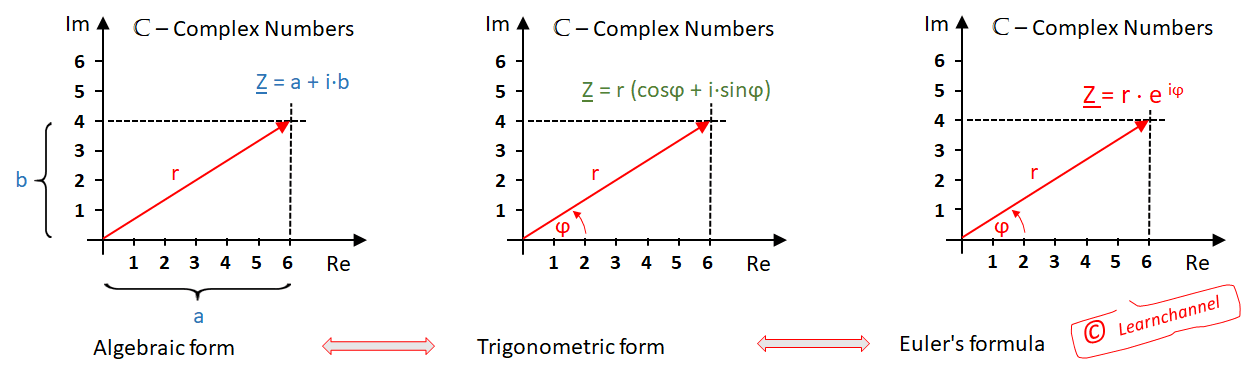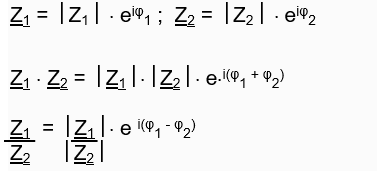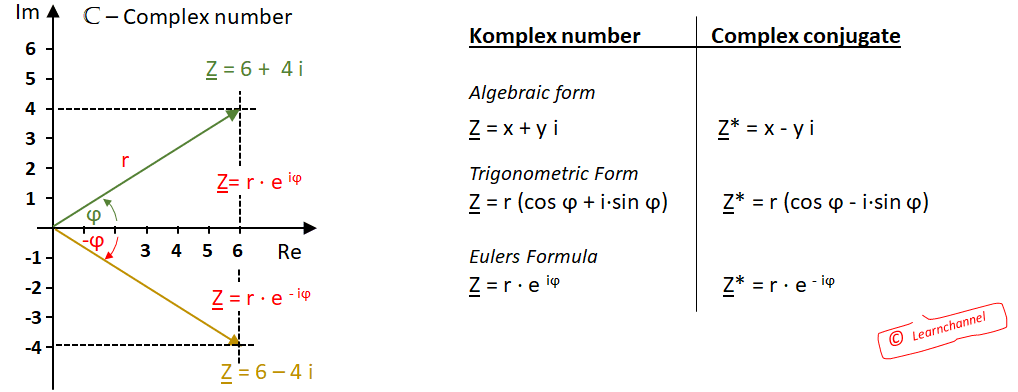# Calculating with complex numbers

Calculating with Complex numbers

### Representations for Complex Numbers

There are three types of representation for complex numbers: The component form, the trigonometric form, and the Exponential form, with all their advantages and disadvantages. Here you will learn how to convert complex numbers into one type of representation.Representations for Complex Numbers

### Convert the component form into trigonometric form:

Ι Z Ι = r = (x2 + y2)

with x = r cosϕ and y = r sinϕ

=>  Z = r (cosϕ + i · sinϕ)  and φ = arctan (y/x) the x- and y-coordinates are clearly defined.

### Derivation of Euler's form for complex numbers:

MacLaurin's series for eϕ:  eϕ  = 1+ φ + φ2 + φ3 + φ4  +…
.                                                       1!    2!     3!     4!

Replace φ for j·φ and you get:

ejϕ  = 1+ + (jφ)2 + (jφ)3 + (jφ)4  +…  = 1+ - φ2 - jφ3 + φ4 +…  =
.              1!      2!        3!       4!                     1!    2!     3!     4!

ejϕ  =  1 -  φ2  + φ4  +  j (  φ  -  φ3 + φ5 -…  )
2!      4!                   3!     5!
|_________|           |___________|
cos φ                    sin φ    (according to the definition of the sine and cosine series)

=>  ejϕ  = cos φ + j sinφ

or with consideration of the length of the pointer follows:     Z = r × e iϕ

### Addition and subtraction of complex numbers

The addition and subtraction of complex numbers is most easily done with the Algebraic form. Simply add or subtract their real and imaginary parts:

Z1 = a + i·b     =>   Z1 + Z2 = (a + c) + i (b + d)

Z2 = c + i·d            Z1 - Z2  = (a - c) + i (b - d)

### Multiplication and division of complex numbers

The easiest way to multiply or divide complex numbers is to use the exponential formula or Eulers form. Here, when multiplying, the amounts must be multiplied and the angles added. In division, the amounts are divided and the angles are subtracted:Multiplication and division of complex numbers

### The complex conjugate

You get the complex conjugate simply by changing the sign of the imaginary part of the complex number. It corresponds to a mirroring of the pointer on the real axis.The complex conjugate

Note: Multiplying a complex number by its complex conjugate gives a real number. This allows complex parts to be removed from a system of equations.

Mnemonics

• For complex numbers, the terms 'greater than' or 'less than' are not defined.
• Two complex numbers are equal if their real and imaginary parts are equal.
• A complex number with the imaginary part equal to zero is a member of the real numbers.
• A complex number with zero real part is a member of the imaginary numbers.
• Two complex numbers are complex conjugate if they differ only in the sign of the imaginary part.